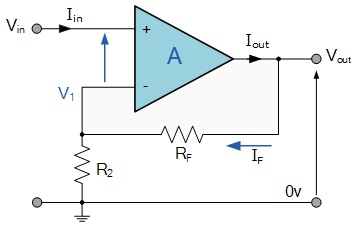Non - Inverting Amplifier

Theory

Op-amp Non - Inverting Amplifier CircuitFig.1 Non - Inverting Amplifier Circuit

To understand how the non-inverting amplifier circuit works, we need to derive a relationship between the input voltage Vin and the output voltage Vout. For an ideal op-amp, there is no loading effect at the input, so

Vp = Vi

Since the current flowing into the inverting input of an ideal op-amp is zero, the current flowing through R1 is equal to the current flowing through R2 (by Kirchhoff’s Current Law -- which states that the algebraic sum of currents flowing into a node is zero - to the inverting input node). We can therefore apply the voltage-divider formula find Vn:We know that Vin = Vp = Vn, so